# ISTEXT Excel FunctionArticle byExcelMojo Team## What Is ISTEXT Function In Excel?

The ISTEXT function in Excel is an inbuilt Information function. It returns TRUE if the given value is a text string and FALSE otherwise. Users can use the ISTEXT Excel function to perform tasks such as ensuring the entered value in a cell is text and checking if a cell contains the specified text. And the function is useful for highlighting cells containing text values.

For example, column A in the below table contains a set of data, and column B shows the formulas used to enter each data value in column A.

Suppose the requirement is to check whether the data values in the cell range A2:A9 are text and display the results in column C. Then, according to the ISTEXT Excel function definition, we can apply the ISTEXT() in the target cells to get the required output.

In the above ISTEXT Excel function example, the function accepts a column A cell value as input. It returns TRUE if the value is text and FALSE otherwise.

Cells A2 and A7:A9 contain text values, and hence the ISTEXT Excel function return value in each corresponding target cell is TRUE. The data entered in cell A9 is a number with an apostrophe in the front, thus making it a text value.

On the other hand, the cell A6 data is a logical value. And hence the ISTEXT Excel function returns FALSE. And for cells containing data other than text or formulas returning values that are not text, the ISTEXT() output is FALSE.

###### Key Takeaways
• The ISTEXT Excel Function checks if the given value is text. And if the condition holds, the function returns TRUE and FALSE otherwise.
• The ISTEXT function is useful for highlighting cells with text values, checking if a cell contains a particular text, and ensuring the data entered in a cell is text.
• The ISTEXT function accepts one mandatory argument, value, as input.
• Using ISTEXT() with other Excel functions and features, such as IF, SUMPRODUCT, Conditional Formatting, and Data Validation, gives excellent outcomes.

### ISTEXT() Excel Formula

The ISTEXT Excel function syntax is:

where,

• value: The value or expression we require to check if it is text using the ISTEXT Excel function. And it is a mandatory argument.

Further, below are a few critical aspects of the ISTEXT() you must know to use the function properly.

• We can supply a blank cell, error, text, number, logical value, or a cell reference to the given value as the value argument.
• The ISTEXT Excel function return value will be FALSE for logical values (TRUE and FALSE), numeric data, and error values such as #VALUE!.

### How To Use ISTEXT Excel Function?

The steps to use the ISTEXT Excel function are as follows:

1. First, ensure the value we require to test is accurate and has the required data format.
2. Next, select the required target cell and enter the ISTEXT().
3. Finally, press Enter to view the check result.

The below example explains the steps mentioned above to apply the ISTEXT Excel function.

Consider the following table containing data values of different data formats.

Suppose we must check if the specified data values in column A are text and show the output in column C. Then applying the ISTEXT() in the target cells can fetch us the required data.

1. Select the target cell C2, enter ISTEXT(), and press Enter.

=ISTEXT(A2)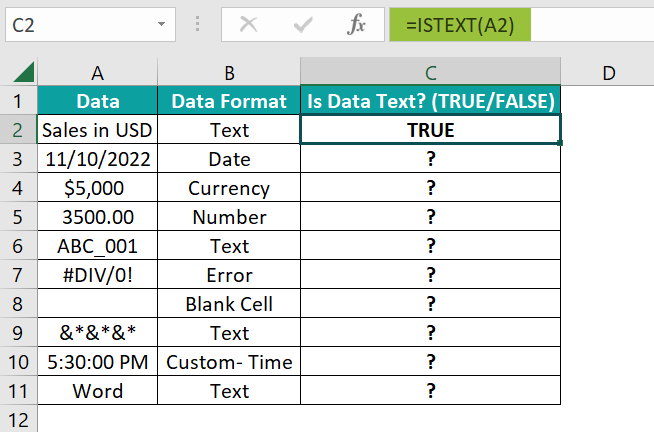Entering the value directly as the function argument will give the same result.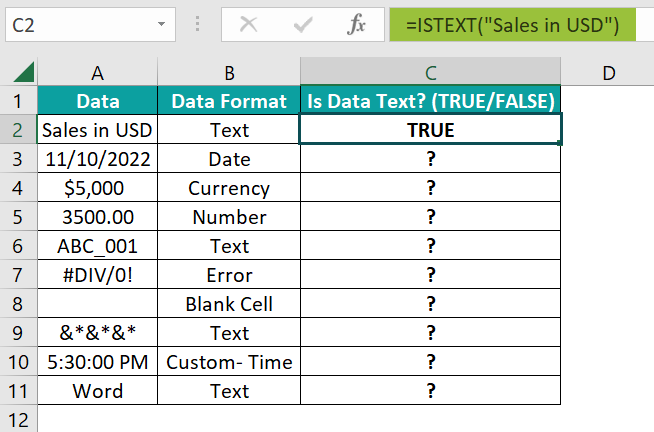Here, the value supplied to the ISTEXT() is in double quotes as the cell A2 data format is Text. The double quotations are not required while providing values of other data formats as the ISTEXT function argument.

Alternatively, we can use the ISTEXT Excel Function Arguments dialog box to apply the ISTEXT(). And for that, we must select the target cell and go to FormulasMore FunctionsInformationISTEXT to open the Function Arguments window.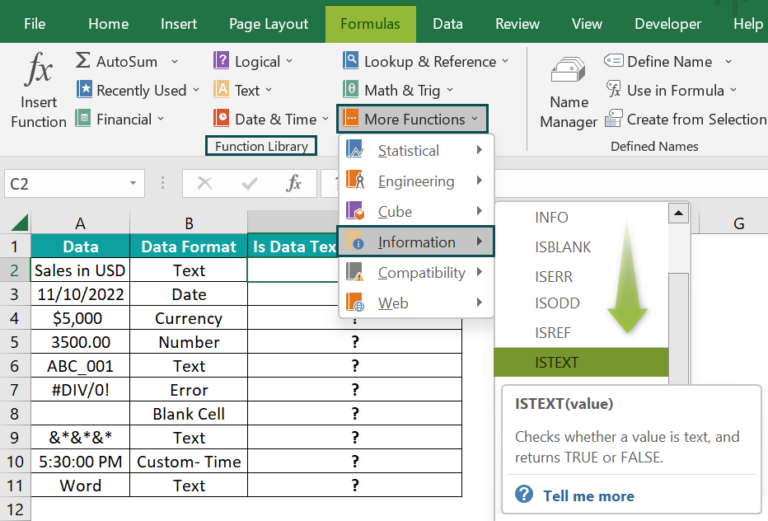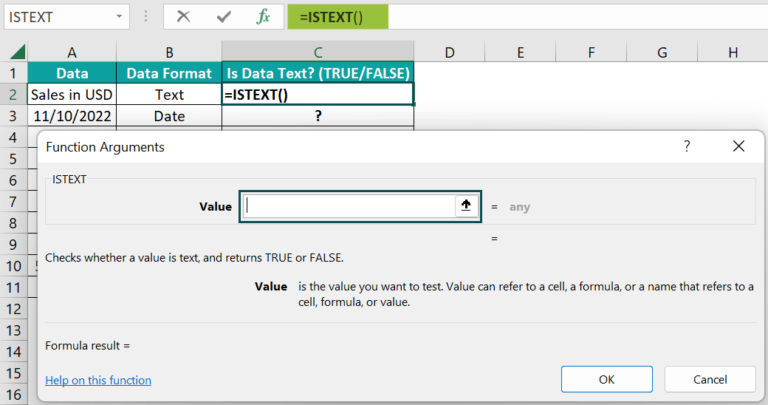Next, enter the value to test for text in the Value field and click OK in the ISTEXT Excel Function Arguments dialog box to view the result in the target cell.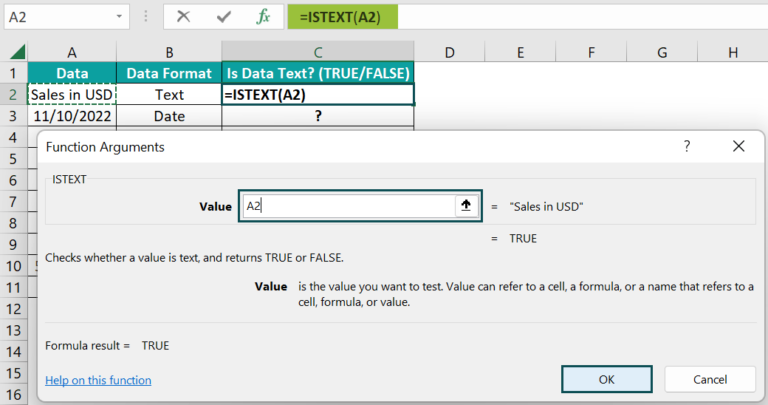2. Apply the formula in cell range C3:C11 using the fill handle.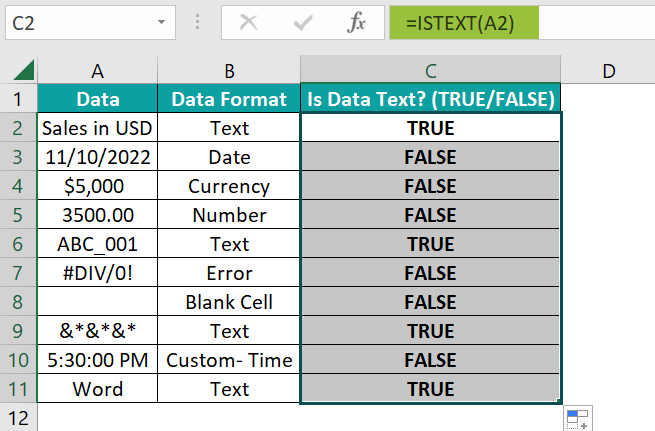In the above ISTEXT Excel function example, cells A2, A6, A9, and A11 contain text values. And thus, the function returns TRUE in the corresponding target cells.

On the other hand, the ISTEXT() output is FALSE in target cells where the supplied data is not in the text format.

### Examples

Check out the below examples to understand the ISTEXT Excel function definition and apply the function in the best way possible.

#### Example #1

This example shows how to use the ISTEXT Excel function to count the number of cells containing text values in a given cell range.

The table below shows a list of model names.

Suppose we need to count the number of cells in the cell range A2:A9 that contain text data and display the output in cell B11. Then, we can use the ISTEXT() with SUMPRODUCT excel function in the target cell to get the required data.

• Step 1: Select the target cell B11, enter the below formula, and press Enter.

=SUMPRODUCT(ISTEXT(A2:A9)*1)

The ISTEXT() checks the cells in the cell range A2:A9 for text data to return the result as an array of TRUEs and FALSEs, {FALSE;TRUE;FALSE;TRUE;FALSE;TRUE;FALSE;TRUE}.

Next, multiplying the above ISTEXT function output with the value 1 converts the TRUE and FALSE values into ones and zeros, {0;1;0;1;0;1;0;1}. Finally, the SUMPRODUCT() adds the resulting array values to return the total cells count with text values as 4.

#### Example #2

We shall see how the ISTEXT Excel function helps validate and ensure the user enters only text in the required cell.

The table below contains a customer satisfaction survey questionnaire and a feedback column.

Suppose the requirement is to ensure the customer only enters text in the feedback column. Then, here is how we can apply the ISTEXT Excel function in the target cells using the Data Validation excel feature in the Data tab.

• Step 1: Select the cell range B2:B6, which is the range to validate, and click DataData Validation to open the Data Validation window.
• Step 2: In the Settings tab, set the Allow field as Custom using the option in the drop-down list.

Next, enter the ISTEXT() in the Formula field to set the data validation condition.

• Step 3: Go to the Error Alert tab to update the Title and Error message fields.

Step 4: Finally, click OK to close the window.

• Step 5: Let us see how the data validation occurs. Assume the customer enters the feedback in cell B2.

As the entered value is a text, the feedback gets accepted.

• Step 6: Next, the customer enters his feedback about the store hours as 12. But Excel shows an error message, Invalid Entry, and asks the customer to enter a valid text. The reason is that the entered feedback is not text data but a number.

Click Retry to enter a valid text as the feedback.

Likewise, the customer can fill in the remaining data. And the data validation feature will ensure they enter only text in the column B cells due to the ISTEXT().

#### Example #3

We can use the ISTEXT Excel function as a conditional formatting criterion.

For example, the table below contains a list of students and their grades.

Consider the requirement to highlight the column B cells in the above table containing text values (grades). Then, here is how we can use the ISTEXT Excel function as a conditional formatting criterion to highlight the required cells.

• Step 1: Select the cell range B2:B11 and go to HomeConditional FormattingNew Rule to open the New Formatting Rule window.
• Step 2: Choose the last Rule Type to enter the criterion and set the format required to highlight cells containing text values.
• Step 3: Once we click the Format option, the Format Cells window will open.

Go to the Fill tab, pick the color we wish to use to highlight the required cells, and click OK.

• Step 4: Finally, click OK in the New Formatting Rule window to close it and view the highlighted cells.

The ISTEXT() checks the cells in the range B2:B11 for text values, and the condition holds in cells B2, B4, B6:B9, and B11. So, they get highlighted, leaving out the blank cell, error, and percentage values, where the ISTEXT() returns FALSE.

### Important Things To Note

• The ISTEXT Excel function returns only logical values, TRUE or FALSE.
• The ISTEXT function can take a text, numeric data, logical value, error, cell references to these values, or a blank cell as the value argument.
• The ISTEXT function returns FALSE if the supplied data is a logical, numeric, or error value. However, supplying such values in double quotations will ensure the ISTEXT function returns TRUE.

1. Where is the ISTEXT function in Excel?

The ISTEXT function in Excel is in the Formulas tab. Click FormulasMore FunctionsInformationISTEXT to access it.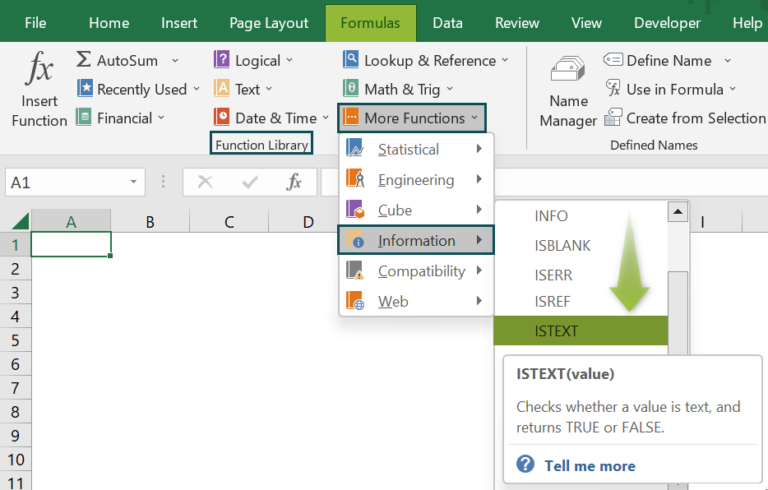2. How to apply the ISTEXT Excel function with IF()?

We can apply the ISTEXT Excel function with IF() in the following way. Let us see the steps with an example.

Consider the table below contains a list of the latest smartphone models.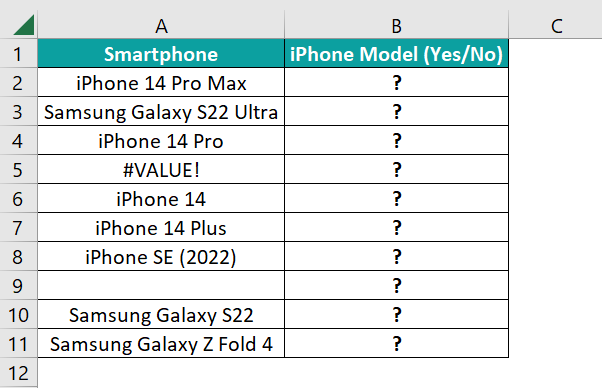Suppose the requirement is to determine if the column A cells contain text values and then check if the specific smartphone is an iPhone model. And we need to display the results in column B.

Then, here is how we can use the ISTEXT() with the IF() in the target cells and get the required data.

• Step 1: Select the target cell B2, enter the below formula, and press Enter.
=IF(ISTEXT(A2),IF(COUNTIF(A2,”*iPhone*”),”Yes”,”No”),””)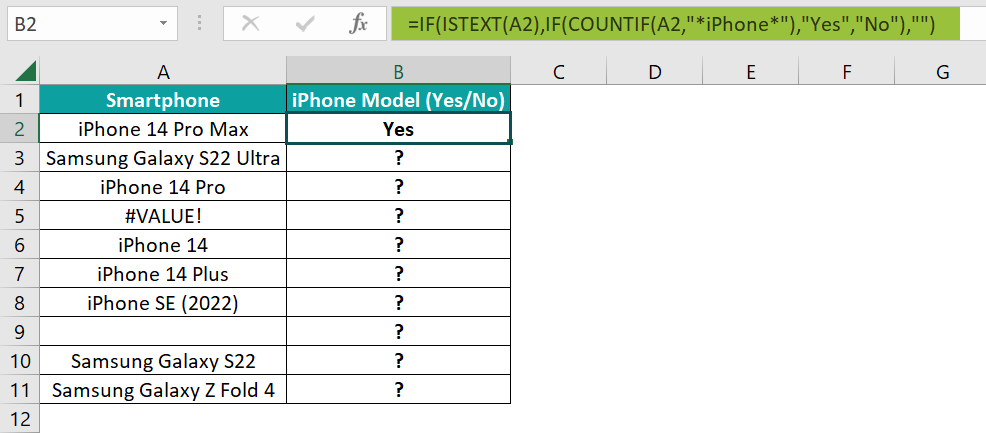• Step 2: Using the fill handle, copy the formula in cells B3:B11.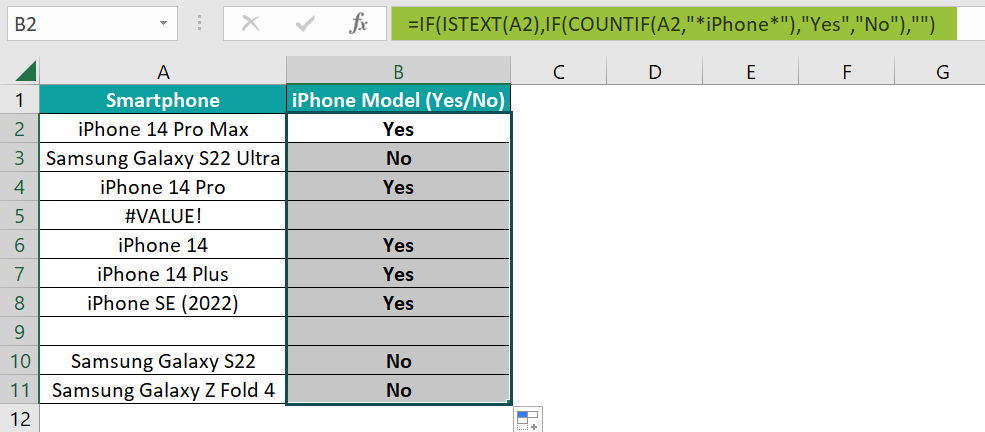Let us consider the cell B11 expression to check how the formula works. First, the ISTEXT() checks if cell A11 contains text value and returns TRUE. So, as the outer IF() condition holds, the inner IF() gets executed. But as the cell A11 value does not contain the phrase “iPhone”, the COUNTIF() returns 0. So, the IF condition is FALSE, and the inner IF() returns No.

On the other hand, as cell A5 contains an error value and cell A9 is blank, the ISTEXT() within the outer IF() returns FALSE. And thus, the outer IF() output is a blank in the corresponding target cells, B5 and B9.

3. How to apply the ISTEXT function in Excel VBA?

We can apply the ISTEXT function in Excel VBA using the below method.

Application.WorksheetFunction.IsText(value)

The argument value in the above method has the same meaning as the ISTEXT function argument explained in the ISTEXT() Excel Formula section of the above article.